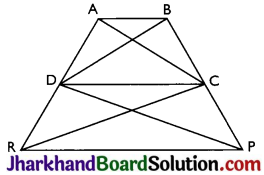# JAC Class 9 Maths Solutions Chapter 9 Areas of Parallelograms and Triangles Ex 9.3

Jharkhand Board JAC Class 9 Maths Solutions Chapter 9 Areas of Parallelograms and Triangles Ex 9.3 Textbook Exercise Questions and Answers.

## JAC Board Class 9th Maths Solutions Chapter 9 Areas of Parallelograms and Triangles Ex 9.3

Page-162

Question 1.
In Fig, E is any point on median AD of a ∆ABC. Show that ar(∆ABE) = ar(∆ACE).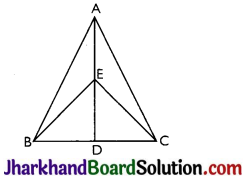Given, AD is median of ∆ABC. Thus, it will divide ∆ABC into two triangles of equal area.
∴ ar(ABD) = ar(ACD) …(i)
Also, ED is the median of ∆EBC.
∴ ar(EBD) = ar(ECD) ,..(ii)
Subtracting (ii) from (i),
ar(ABD) – ar(EBD) = ar(ACD) – ar(ECD)
⇒ ar(ABE) = ar(ACE)

Question 2.
In a triangle ABC, E is the mid-point of median AD. Show that ar(BED) = $$\frac{1}{4}$$ ar(ABC).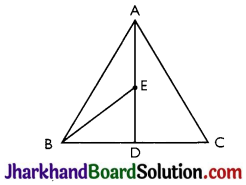In ΔABD, E is a mid-point of AD
⇒ BE is the median
⇒ ar (BED) = $$\frac{1}{2}$$ ar (ABD) ……….(i)
[as median divides triangle into two triangles of equal area]
Also, in ΔABC, AD is the median
⇒ ar (ABD) = $$\frac{1}{2}$$ ar (ABC) ……….(ii)
From (i) and (ii), we get
ar (BED) = $$\frac{1}{2}$$ ar (ABD)
= $$\frac{1}{2}$$ [$$\frac{1}{2}$$ ar (ABC)]
= $$\frac{1}{4}$$ ar (ABC)
Therefore, ar (BED) = $$\frac{1}{4}$$ ar (ABC)Question 3.
Show that the diagonals of a parallelogram divide it into four triangles of equal area.
O is the mid-point of AC and BD. (diagonals of parallelogram bisect each other)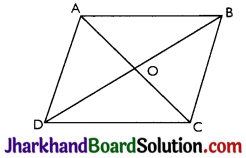In ΔABC, BO is the median.
∴ ar(AOB) = ar(BOC) ……….(i)
Also, in ΔBCD, CO is the median.
∴ ar(BOC) = ar(COD) ………(ii)
In ΔACD, OD is the median.
∴ ar(AOD) = ar(COD) …(iii)
In ΔABD, AO is the median.
∴ ar(AOD) = ar(AOB) …(iv)
From equations (i), (ii), (iii) and (iv),
ar(BOC) = ar(COD) = ar(AOD) = ar(AOB)
So, the diagonals of a parallelogram divide it into four triangles of equal area.

Question 4.
In Fig, ABC and ABD are two triangles on the same base AB. If line segment CD is bisected by AB at O, show that: ar(ABC) = ar(ABD).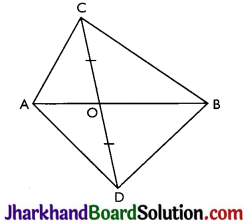AO is the median. (CD is bisected by AB at O)
∴ ar(AOC) = ar(AOD) ………(i)
Also, in ABCD, BO is the median. (CD is bisected by AB at O)
∴ ar(BOC) = ar(BOD) ………(ii)
Adding (i) and (ii) we get,
ar(AOC) + ar(BOC) = ar(AOD) + ar(BOD)
∴ ar(ABC) = ar(ABD)

Page-163

Question 5.
D, E and F are respectively the mid-points of the sides BC, CA and AB of a ∆ABC. Show that
(i) BDEF is a parallelogram
(ii) ar(ΔDEF) = $$\frac{1}{4}$$ ar(ABC)
(iii) ar(BDEF) = $$\frac{1}{2}$$ ar(ABC)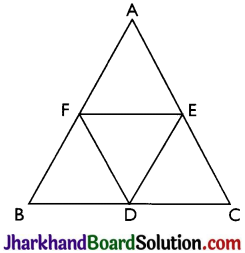(i) In ΔABC,
EF || BC and EF = $$\frac{1}{2}$$ BC (by mid-point theorem)
⇒ EF || BD and EF = $$\frac{1}{2}$$ BC
Also, BD = $$\frac{1}{2}$$ BC (D is the mid-point)
So, BD = EF and EF || BD
Thus, a pair of opposite sides are equal in length and parallel to each other.
∴ BDEF is a parallelogram.

(ii) Similarly, DCEF and AFDE are parallelograms, (as in (i) part)
Diagonal of a parallelogram divides it into two triangles of equal area.
∴ ar(∆BFD) = ar(∆DEF) (For parallelogram BDEF) ………..(i)
Also, ar(∆CDE) = ar(∆DEF) (For parallelogram DCEF) ……..(ii)
ar(∆AFE) = ar(∆DEF) (For parallelogram AFDE) ………….(iii)
From (i), (ii) and (iii)
ar(∆BFD) = ar(∆AFE) = ar(∆CDE) = ar(∆DEF)
⇒ ar(∆BFD) + ar(∆AFE) + ar(∆CDE) + ar(∆DEF) = ar(∆ABC)
⇒ 4 ar(∆DEF) = ar(∆ABC)
⇒ ar(DEF) = $$\frac{1}{4}$$ ar(ABC)

(iii) Area (parallelogram BDEF) = ar(∆DEF) + ar(∆BDF)
⇒ ar(parallelogram BDEF) = ar(∆DEF) + ar(∆DEF) [∵ ar (DEF) = ar (BDF)]
⇒ ar(parallelogram BDEF) = 2 × ar(∆DEF)
⇒ ar(parallelogram BDEF) = 2 × $$\frac{1}{2}$$ ar(∆ABC) [From (ii) part]
⇒ ar(parallelogram BDEF) = $$\frac{1}{2}$$ ar(∆ABC)Question 6.
In Fig, diagonals AC and BD of quadrilateral ABCD intersect at O such that OB = OD. If AB = CD, then show that:
(i) ar(DOC) = ar(AOB)
(ii) ar(DCB) = ar(ACB)
(iii) DA || CB or ABCD is a parallelogram.
[Hint: From D and B, draw perpendiculars to AC.]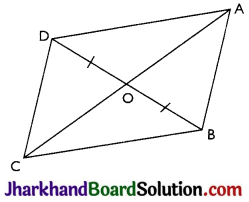Given: OB = OD and AB = CD
Construction: DE ⊥ AC and BF ⊥ AC are drawn.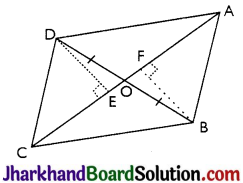Proof:
(i) In ∆DOE and ∆BOF,
∠DEO = ∠BFO (Perpendiculars)
∠DOE = ∠BOF (Vertically opposite angles)
OD = OB (Given)
Therefore, ∆DOE ≅ ∆BOF (by ∆AS congruence criterion)
Thus, DE = BF (By CPCT) ……….(i)

Also, ar(∆DOE) = ar(∆BOF) (Congruent triangles have equal area) ……….(ii)
Now, in ∆DEC and ∆BFA,
∠DEC = ∠BFA (Perpendiculars)
CD = AB (Given)
DE = BF (From (i))
Therefore, ∆DEC ≅ ∆BFA (by RHS congruence criterion)
Thus, ar(∆DEC) = ar(∆BFA) (Congruent triangles have equal area) ………(iii)
⇒ ar(DOC) = ar(AOB)

(ii) ar(∆DOC) = ar(∆AOB)
⇒ ar(∆DOC) + ar(∆DOC) = ar(∆AOB) + ar(∆BOC)
⇒ ar(∆DCB) = ar(∆ACD)

(iii) ar(∆DCB) = ar(∆ACB)
If two triangles are having same base and equal areas, these will be between same parallels
⇒ AB || CD ………..(iv)
So, AB = CD and AB || CD
For quadrilateral ABCD, a pair of opposite sides is equal and parallel.
Therefore, ABCD is parallelogram.

Question 7.
D and E are points on sides AB and AC respectively of ∆ABC such that ar(DBC) = ar(EBC). Prove that DE || BC.
ADBC and AEBC are on the same base BC and also having equal areas. Therefore, they will lie between the same parallel lines.
Thus, DE || BC.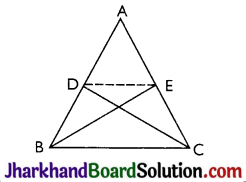Question 8.
XY is a line parallel to side BC of a triangle ABC. If BE || AC and CF || AB meet XY at E and F respectively, show that: ar(∆ABE) = ar(∆ACF)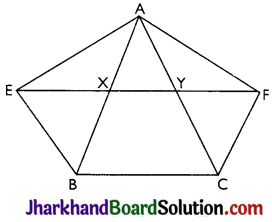Given: XY || BC, BE || AC and CF || AB
To show: ar(∆ABE) = ar(∆ACF)
Proof: EY || BC (XY||BC) ……..(i)
Also, BE || CY (BE || AC) ……….(ii)
From (i) and (ii),
BEYC is a parallelogram. (Both the pairs of opposite sides are parallel.)
Similarly, BXFC is a parallelogram.
Parallelograms on the same base BC and between the same parallels EF and BC.
⇒ ar(BEYC) = ar(BXFC) (Parallelograms on the same base BC and between the same parallels EF and BC) … (iii)
Also, ∆AEB and parallelogram BEYC are on the same base BE and between the same parallels BE and AC.
⇒ ar(∆AEB) = j ar(BEYC) …(iv)
Similarly, ∆ACF and parallelogram BXFC on the same base CF and between the same parallels CF and AB.
⇒ ar(A ACF) = $$\frac{1}{2}$$ ar(BXFC) …(v)
From (iii), (iv) and (v),
ar(∆AEB) = ar(∆ACF)Question 9.
The side AB of a parallelogram ABCD is produced to any point P. A line through A and parallel to CP meets CB produced at Q and then parallelogram PBQR is completed (see Fig.). Show that: ar(ABCD) = ar(PBQR).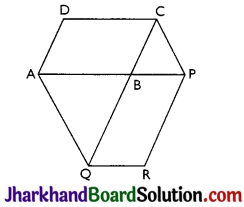[Hint: Join AC and PQ. Now compare ar(ACQ) and ar(APQ).]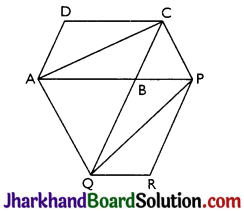AC and PQ are joined.
ar(∆ACQ) = ar(∆APQ) (On the same base AQ and between the same parallel lines AQ and CP)
⇒ ar(∆ACQ) – ar(∆ABQ) = ar(∆APQ) – ar(∆ABQ)
⇒ ar(∆ABC) = ar(∆QBP) …(i)
AC and QP are diagonals of ABCD and PBQR respectively.
Thus,
ar(ABC) = $$\frac{1}{2}$$ ar(ABCD) …(ii)
ar(QBP) = $$\frac{1}{2}$$ ar(PBQR) …(iii)
From (i), (ii) and (iii),
$$\frac{1}{2}$$ar(ABCD) = $$\frac{1}{2}$$ ar(PBQR)
⇒ ar(ABCD) = ar(PBQR)

Question 10.
Diagonals AC and BD of a trapezium ABCD with AB || DC intersect each other at O. Prove that ar(AOD) = ar(BOC).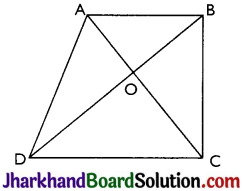∆DAC and ∆DBC lie on the same base DC and between the same parallels AB and CD.
∴ ar(∆DAC) = ar(∆DBC)
⇒ ar(∆DAC) – ar(∆DOC) = ar(∆DBC) – ar(∆DOC)
⇒ ar(∆AOD) = ar(∆BOC)

Question 11.
In Fig, ABCDE is a pentagon. A line through B parallel to AC meets DC produced at F. Show that
(i) ar(ACB) = ar(ACF)
(ii) ar(AEDF) = ar(ABCDE)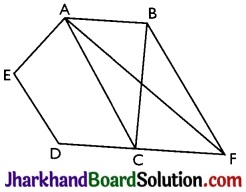(i) ∆ACB and ∆ACF lie on the same base AC and between the same parallels AC and BF.
∴ ar(∆ACB) = ar(∆ACF)

(ii) ar(∆ACB) = ar(∆ACF)
⇒ ar(∆ACB) + ar(∆ACDE) = ar(∆ACF) + ar(∆ACDE)
⇒ ar(ABCDE) = ar(∆AEDF)

Page-164

Question 12.
A villager Itwaari has a plot of land of the shape of a quadrilateral. The Gram . Panchayat of the village decided to take over some portion of his plot from one of the corners to construct a Health Centre. Itwaari agrees to the above proposal with the condition that he should be given equal amount of land in lieu of his land adjoining his plot so as to form a triangular plot. Explain how this proposal will be implemented.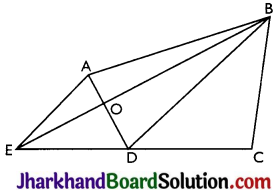Let ABCD be the plot of the land of the shape of a quadrilateral.
Construction:
Diagonal BD is joined. AE is drawn parallel to BD. BE is joined which intersects AD at O. ABCE is the shape of the original field and ∆AOB is the area for constructing health centre. Also, ADEO land joined to the plot.
To prove: ar(∆DEO) = ar(∆AOB)
Proof: ∆DEB and ∆DAB lie on the same base BD and between the same parallel lines BD and AE.
ar(∆DEB) = ar(∆DAB)
⇒ ar(∆DEB) – ar(∆DOB) = ar(∆DAB) – ar(∆DOB)
⇒ ar(∆DEO) = ar(∆AOB)

Question 13.
ABCD is a trapezium with AB || DC. A line parallel to AC intersects AB at X and BC at Y. Prove that ar(ADX) = ar(ACY). [Hint: Join CX.]
Given: ABCD is a trapezium with AB || DC and XY || AC
Construction: CX is joined.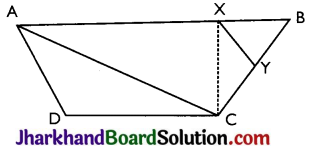(On the same base AX and between the same parallels AB and CD)
Also, ar(A AXC) = ar(A ACY) …(ii)
(On the same base AC and between the same parallels XY and AC.)
From (i) and (ii), ar(∆ADX) = ar(∆ACY)

Question 14.
In Fig, AP || BQ || CR. Prove that: ar(AQC) = ar(PBR).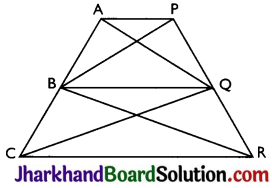Given: AP || BQ || CR
To Prove: ar(AQC) = ar(PBR)
Proof: ar(∆AQB) = ar(APBQ) …(i)
(On the same base BQ and between the same parallels AP and BQ.)
Also, ar(ABQC) = ar(ABQR) …(ii)
(On the same base BQ and between the same parallels BQ and CR.)
ar(∆AQB) + ar(ABQC)
= ar(APBQ) + ar(ABQR)
⇒ ar(A AQC) = ar(A PBR)Question 15.
Diagonals AC and BD of a quadrilateral ABCD intersect at O in such a way that ar(AOD) = ar(BOC). Prove that ABCD is a trapezium.
Given: ar(∆AOD) = ar(ABOC)
To Prove: ABCD is a trapezium.
Proof: ar(∆AOD) = ar(ABOC) (Given)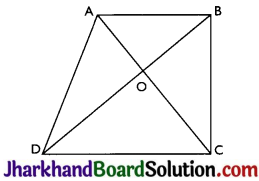= ar(ABOC) + ar(∆AOB)
Areas of ∆ADB and ∆ACB are equal. Therefore, they must lie between the same parallel lines.
Thus, AB || CD
Therefore, ABCD is a trapezium.

Question 16.
In Fig, ar(DRC) = ar(DPC) and ar(BDP) = ar(ARC). Show that both the quadrilaterals ABCD and DCPR are trapeziums.